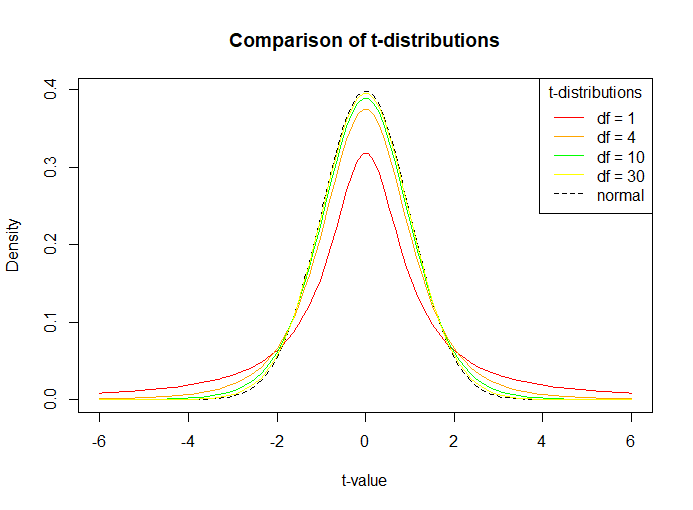Related Articles
Understanding the t-distribution in R
• Last Updated : 24 Feb, 2021

The t-distribution is a type of probability distribution that arises while sampling a normally distributed population when the sample size is small and the standard deviation of the population is unknown. It is also called the Student’s t-distribution. It is approximately a bell curve, that is, it is approximately normally distributed but with a lower peak and more observations near the tail. This implies that it gives a higher probability to the tails than the standard normal distribution or z-distribution (mean is 0 and the standard deviation is 1).

Degrees of Freedom is related to the sample size and shows the maximum number of logically independent values that can freely vary in the data sample. It is calculated as n – 1, where n is the total number of observations. For example, if you have 3 observations in a sample, 2 of which are 10,15 and the mean is revealed to be 15 then the third observation has to be 20. So the Degrees of Freedom, in this case, is 2 (only two observations can freely vary). Degrees of Freedom is important to a t-distribution as it characterizes the shape of the curve. That is, the variance in a t-distribution is estimated based on the degrees of freedom of the data set. As the degrees of freedom increase, the t-distribution will come closer to matching the standard normal distribution until they converge (almost identical). Therefore, the standard normal distribution can be used in place of the t-distribution with large sample sizes.

A t-test is a statistical hypothesis test used to determine if there is a significant difference (differences are measured in means) between two groups and estimate the likelihood that this difference exists purely by chance (p-value). In a t-distribution, a test statistic called t-score or t-value is used to describe how far away an observation is from the mean. The t-score is used in t-tests, regression tests and to calculate confidence intervals.

## Student’s t-distribution in R

Functions used:

• To find the value of probability density function (pdf) of the Student’s t-distribution given a random variable x, use the dt() function in R.

Syntax: dt(x, df)

Parameters:

• x is the quantiles vector
• df is the degrees of freedom
• pt() function is used to get the cumulative distribution function (CDF) of a t-distribution

Syntax: pt(q, df, lower.tail = TRUE)

Parameter:

• q is the quantiles vector
• df is the degrees of freedom
• lower.tail – if TRUE (default), probabilities are P[X ≤ x], otherwise, P[X > x].
• The qt() function is used to get the quantile function or inverse cumulative density function of a t-distribution.

Syntax: qt(p, df, lower.tail = TRUE)

Parameter:

• p is the vector of probabilities
• df is the degrees of freedom
• lower.tail – if TRUE (default), probabilities are P[X ≤ x], otherwise, P[X > x].

### Approach

• Set degrees of freedom
• To plot the density function for student’s t-distribution follow the given steps:
• First create a vector of quantiles in R.
• Next, use the dt function to find the values of a t-distribution given a random variable x and certain degrees of freedom.
• Using these values plot the density function for student’s t-distribution.
• Now, instead of the dt function, use the pt function to get the cumulative distribution function (CDF) of a t-distribution and the qt function to get the quantile function or inverse cumulative density function of a t-distribution. Put it simply, pt returns the area to the left of a given random variable q in the t-distribution and qt finds the t-score is of the pth quantile of the t-distribution.

Example: To find a value of t-distribution at x=1, having certain degrees of freedom, say Df = 25,

## R

 `# value of t-distribution pdf at ``# x = 0 with 25 degrees of freedom``dt``(x = 1, df = 25)`

Output:

`0.237211`

Example:

Code below shows a comparison of probability density functions having different degrees of freedom. It is observed as mentioned before, larger the sample size (degrees of freedom increasing), the closer the plot is to a normal distribution (dotted line in figure).

## R

 `# Generate a vector of 100 values between -6 and 6``x <- ``seq``(-6, 6, length = 100)`` ` `# Degrees of freedom``df = ``c``(1,4,10,30)``colour = ``c``(``"red"``, ``"orange"``, ``"green"``, ``"yellow"``,``"black"``)`` ` `# Plot a normal distribution``plot``(x, ``dnorm``(x), type = ``"l"``, lty = 2, xlab = ``"t-value"``, ylab = ``"Density"``, ``     ``main = ``"Comparison of t-distributions"``, col = ``"black"``)`` ` `# Add the t-distributions to the plot``for ``(i ``in` `1:4){``  ``lines``(x, ``dt``(x, df[i]), col = colour[i])``}`` ` `# Add a legend``legend``(``"topright"``, ``c``(``"df = 1"``, ``"df = 4"``, ``"df = 10"``, ``"df = 30"``, ``"normal"``), ``       ``col = colour, title = ``"t-distributions"``, lty = ``c``(1,1,1,1,2))`

Output:Example: Finding p-value and confidence interval with t-distribution

## R

 `# area to the right of a t-statistic with ``# value of 2.1 and 14 degrees of freedom``pt``(q = 2.1, df = 14, lower.tail = ``FALSE``)`

Output:

`0.02716657`

Essentially we found the one-sided p-value, P(t>2.1) as 2.7%. Now suppose we want to construct a two-sided 95% confidence interval. To do so, find the t-score or t-value for 95% confidence using the qt function or the quantile distribution.

Example:

## R

 `# value in each tail is 2.5% as confidence is 95%``# find 2.5th percentile of t-distribution with ``# 14 degrees of freedom``qt``(p = 0.025, df = 14, lower.tail = ``TRUE``)`

Output:

`-2.144787`

So, a t-value of 2.14 will be used as the critical value for a confidence interval of 95%.

Attention reader! Don’t stop learning now. Get hold of all the important DSA concepts with the DSA Self Paced Course at a student-friendly price and become industry ready.

My Personal Notes arrow_drop_up